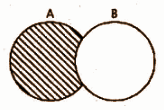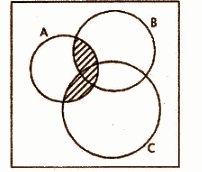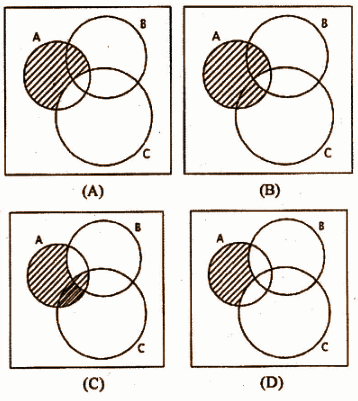# RBSE Maths Class 11 Chapter 1: Important Questions and Solutions

RBSE Maths Chapter 1 – Set theory Class 11 Important questions and solutions can be accessed here. The important questions and solutions of Chapter 1, available at BYJU’S, contain detailed step by step explanations. All these important questions are based on the new pattern prescribed by the RBSE. Students can also get the syllabus and textbooks on RBSE Class 11 solutions.

Chapter 1 of the RBSE Class 11 Maths will help the students to solve problems related to sets and their representation, different types of sets, subset, operations on sets, representation of initial operations on sets by Venn diagram.

### RBSE Maths Chapter 1: Exercise 1.1 Textbook Important Questions and Solutions

Question 1: Fill in the blanks with appropriate symbols ∈ or ∉ to make the following statements correct:

(i) 3 _____ {1, 2, 3, 4, 5}

(ii) 2.5 _____ N

(iii) 0 _____ Q

Solution:

(i) ∈

(ii) ∉

(iii) ∈

Question 2. Fill the blanks with the symbols ⊂ or ⊄ to make the following statements correct:

(i) {2, 3, 4, 5} _____ {1, 2, 3, 4, 5}

(ii) {a, e, o} _____ {a, b, c}

(iii) {z : z is an equilateral triangle in a plane} _____ {z : z is a triangle in a plane}

(iv) {x : x is a natural number} _____ {x : x is an odd whole number}

Solution:

(i) {2, 3, 4, 5} ⊂ {1, 2, 3, 4, 5}

(ii) {a, e, o} ⊄ {a, b, c}

(iii) Many triangles are possible on a plane.

So, {z: z is an equilateral triangle in a plane} ⊂ (z: z is a triangle in a plane}

(iv) The first set is (1, 2, 3, 4,….} and second set is {…… -3, -1, 0, 1, 3, 5, . . }.

So, (x : x is a natural number} ⊄ {x : x is an odd whole number}.

Question 3. Examine the following statements:

(i) {c, b} ⊂ {b, a, c]

(ii) {i, e} ⊂ (x : x is vowel of English alphabet}

(iii) {1, 2, 3} ⊄ {1, 3, 2, 5}

(iv) {x : x is an even natural number less than or equal to 4} ⊄ {x : x is a natural number which divides 64}

Solution:

(i) {c, b} ⊂ {b, a, c}

The elements of the first set (c, b) are present in the second set {b, a, c}.

So, the statement is true.

(ii) {i, e} ⊂ {x : x is vowel of English alphabet}

The second set is {a, e, i, o, u}.

The elements of the first set (i, e) is present is the second set {a, e, i, o, u}.

So, the statement is true.

(iii) {1, 2, 3} ⊄ {1, 3, 2, 5}

The elements of the first set {1, 2, 3} are present in the elements of the second set {1, 3, 2, 5}.

So, the statement is false.

(iv) {x : x is an even natural number less than or equal to 4} ⊂ (x : x is a natural number which divides 64}

{2, 4} ⊂ {1, 2, 4, 8, 16, 32, 64}

So, the statement is true.

Question 4. Write power sets of the following:

(i) {a}

(ii) {1, 2, 3}

(iii) {a, b}

(iv) Φ

Solution:

(i) {a}

The subsets of {a} are Φ and {a}.

The power set P{a} = {Φ, {a}}

(ii) {1, 2, 3}

The subsets of {1, 2, 3} are Φ, {1}, {2}, {3}, {1, 2}, {1, 3}, {2, 3} and {1, 2, 3}.

The power set P{1, 2, 3} = {Φ, {1}, {2}, {3}, {1, 2}, {1, 3}, {2, 3}, {1, 2, 3}}

(iii) {a, b}

The subsets of {a, b} are Φ, {a}, {b}, {a, b}

The power set P{a, b} = {Φ, {a}, {b}, {a, b}}

(iv) Φ

Φ is the null set, then the power set P(Φ) = {Φ}

Question 5: Write the following as intervals.

(i) {x : x ∈ R, -3 < x < 6}

(ii) {x : x ∈ R, -4 ≤ x ≤ 8}

(iii) {x : x ∈ R, 4 < x ≤ 9}

(iv) {x : x ∈ R, -6 ≤ x < -1}

Solution:

(i) {x : x ∈ R, -3 < x < 6}

-3 < x < 6 is an open interval where -3 and 6 are not included.

So, {x : x ∈ R, -3 < x < 6} has an interval (-3, 6).

(ii) {x : x ∈ R, -4 ≤ x ≤ 8}

-4 ≤ x ≤ 8 is a closed interval in which (-4 and 8) are included.

So, the interval is [-4, 8].

(iii) {x : x ∈ R, 4 < x ≤ 9}

4 < x ≤ 9 is a semi closed interval where the interval is less than 4 and equal to 9.

So, {x : x ∈ R, 4 < x ≤ 9} has interval (4, 9].

(iv) {x : x ∈ R, -6 ≤ x < -1}

-6 ≤ x < -1 is a semi closed interval where the interval is equal to -6 and less than -1.

Hence, {x : x ∈ R, – 6 ≤ x < -1} has interval [-6, -1).

Question 6. Write the following set in builder form.

(i) (-4, 0)

(ii) [6, 8]

(iii) [-3, 7)

(iv) (3, 10]

Solution:

(i) (-4, 0) = {x : x ∈ R, -4 < x < 0}

(ii) [6, 8] = {x : x ∈ R, 6 ≤ x ≤ 8}

(iii) [- 3, 7) = {x : x ∈ R, -3 ≤ x < 7}

(iv) (3, 10] = (x : x ∈ R, 3 < x ≤ 10}

Question 7: A = {1, 3, 5}, B = {1, 4, 6} and C = {2, 4, 6, 8}, then which of the following may be considered as an universal set?

(i) {0, 1, 2, 3, 4, 5, 6}

(ii) (1, 2, 3, 4, 5, 6, 7, 8}

(iii) {0, 1, 2, 3, 4, 5, 6, 7, 8, 9, 10}

(iv) Φ

Solution:

For set A = {1, 3, 5}, B = {1, 4, 6} and C = {2, 4, 6, 8} we have (ii) and (iii) universal set, because it contains all the elements of the three given sets A, B and C.

So, the options (ii) and (iii) are correct.

### RBSE Maths Chapter 1: Miscellaneous Exercise Important Questions and Solutions

Question 1: The solution of equation x2 + x – 2 = 0 in roster form is

[i] {1, 2}

[ii] {-1, 2}

[iii] {-1, -2}

[iv] {1, -2}

Solution:

The given equation is x2 + x – 2 = 0.

x2 + 2x – x – 2 = 0

x (x + 2) – 1 (x + 2) = 0

(x – 1) (x + 2) = 0

x = 1 or -2

Hence, option [iv] is correct.

Question 2: {B: Y: y is a vowel of the English alphabet}, its roster form is:

[i] {a, e, i, o}

[ii] {a, o, u}

[iii] {a, e, o, u}

[iv] {a, e, i, o, u}

Solution:

The vowels of the English alphabets are a, e, i, o, u.

The set of English alphabets is {a, e, i, o, u}.

So, option [iv] is correct.

Question 3: Set builder form of set A = (1, 4, 9, 16, 25,…..) will be:

[i] {x : x is an odd natural number}

[ii] {x : x is an even natural number}

[iii] {x : x is square of natural number}

[iv] {x : x is a prime natural no.)

Solution:

Elements of set A = (1, 4, 9,16, 25, …….) are the squares of 1, 2, 3, 4, 5….. respectively.

So, option [iii] is correct.

Question 4: Which of the following sets are infinite in nature?

[i] {x : x ∈ N and (x – 1) (x – 2) = 0}

[ii] {x : x ∈ N and x2 = 4}

[iii] {x : x ∈ N and 2x – 1 = 0)

[iv] {x : x ∈ N and x is a prime number)

Solution:

The choice [iv] consists of a set of prime numbers which are infinite. Hence, the set is infinite.

Question 5: If A = {0}, B = {x : x > 15 and x < 5}, C = {x : x – 5 = 0}, D = {x : x2 = 25}, E = {x : x is a positive integer root of equation x2 – 2x – 15 = 0}, then pair of equal sets is:

[i] A, B

[ii] B, C

[iii] A, C

[iv] C, E

Solution:

Option [iv] is correct. The tabular form of the set C = {5} and set E = {5}

Hence, C = E

So, the pair of equal sets are C and E.

Question 6: For sets Φ, A = {1, 3}, B = {1, 5, 9}, C = {1, 5, 7, 9} the right relation is:

[i] A ⊂ B

[ii] B ⊂ C

[iii] C ⊂ B

[iv] A ⊂ C

Solution:

The choice [ii] is correct. All the elements of set B = {1, 5, 9} is present in set C = (1, 5, 7, 9}

Hence, B ⊂ C.

Question 7: If A = {2, 4, 6, 8} and B = {1, 4, 7, 8} then A – B and B – A will respectively be

[i] {2, 6}; {1, 7}

[ii] {1, 7}; {4, 8}

[iii] {1, 7}; {2, 6}

[iv] {4, 8}; {1, 7}

Solution:

A = {2, 4, 6, 8}

B = {1, 4, 7, 8}

A – B = {2, 6}

B – A = {1, 7}

So, option [i] is correct.

Question 8: Which of the following statements is true?

[i] A ∩ B = Φ ⇒ A = Φ and B = Φ

[ii] A – B = Φ ⇒ A ⊂ B

[iii] A ⋃ B = Φ ⇒ A ⊂ B

[iv] None of these

Solution:

The choice [i] is correct because, in A ∩ B = Φ, Φ is common to both the sets A and B.

Question 9: If A ∩ B = Φ, then:

[i] A – B = Φ

[ii] A – B = A

[iii] A ⋃ B = Φ

[iv] A – B = B

Solution:

It is given that A ∩ B = Φ.

Φ is common in both the sets A and B.

So, the other elements of A, are not present in B.

Hence, A – B = A

The choice [ii] is correct.

Question 10: The shaded part of the following Venn diagram represents[i] A ⋃ B

[ii] A ∩ B

[iii] A – B

[iv] B – A

Solution:

The shaded portion in the figure above represents the elements in A which are not in B.

It is A – B.

So, option [iii] is correct.

Question 11: If A = (1, 2, 3, 4, 5, 6} and B = {2, 4, 6, 8}, then A – B will be:

[i] {1, 3, 5, 8}

[ii] {1, 3, 5}

[iii] {1, 2, 3, 4, 5, 6, 8}

[iv] {0}

Solution:

A = (1, 2, 3, 4, 5, 6} and B = {2, 4, 6, 8}

The set which consists of all the elements of set A, which are not present in set B is A – B.

So, A – B = {1, 3, 5}

The choice [ii] is correct.

Question 12: Which of the following statements is true?

[i] {2, 3, 4, 5} and {3, 6} are disjoint sets.

[ii] {a, e, i, o, u) and (a b, c, d) are disjoint sets.

[iii] {2, 6, 10, 14} and {3, 7, 11,15} are disjoint sets.

[iv] {2, 7, 10} and (3, 7, 11) are disjoint sets.

Solution:

The right answer is choice [iii] because there are no common elements in the two sets.

Question 13: If U = {1, 2, 3, 4, 5, 6}, A = {2, 3} and B = {3, 4, 5} then

[i] (A ⋃ B)’ = {2, 3, 4, 5}

[ii] B – A = {4, 5}

[iii] A – B = {2, 4, 5}

[iv] A ⋃ B = {3}

Solution:

The set which consists of all the elements of set B, which are not present in set A is B – A.

B – A = {4, 5}

Hence, choice [ii] is correct.

Question 14: The shaded portion of the following Venn diagram represents[i] (A ∩ B) ∩ C

[ii] (A ⋃ B) ∩ C

[iii] (A ∩ B) ⋃ C

[iv] (A ∩ B) ⋃ (A ∩ C)

Solution:

In the figure, the first shaded part is common of both the sets A and B that is A B and the second shaded part is common of A and C both that is A C and full shaded region means the union of A B and A C that is (A B) (A C).

So, option [iv] is correct.

Question 15: Which of the following Venn diagrams represents the set A – (B ∩ C)?Solution:

The set which represents all the elements of set A, which are not present in set (B ∩ C) is A – (B ∩ C).

Hence, choice [B] is the correct answer.

Question 16: Write the following sets in roster form.

[i] A = {x: x ∈ N, 2 ≤ x ≤ 9}

[ii] B = {x: x is a two digit number whose sum of the digits is 6}

[iii] C = {set of all the letters of the word MATHEMATICS}

[iv] D = {x: x is a prime number less than 50}

Solution:

[i] A = {x: x ∈ N, 2 ≤ x ≤ 9}

Roster form A = {2, 3, 4, 5, 6, 7, 8, 9}

[ii] B = {x: x is a two digit number whose sum of the digits is 6}

The two-digit natural numbers whose sum of the digits are 6 = 15, 24, 33, 42, 51.

Roster form B = {15, 24, 33, 42, 51}.

[iii] C = {set of all the letters of the word MATHEMATICS}

Roster form of C = {M, A, T, H, E, I, C, S}

[iv] D = {x: x is a prime number less than 50}

The prime numbers which are less than 50 are

2, 3, 5, 7, 11,13, 17,19, 23, 29, 31, 37, 41, 43, 47.

Roster form of C = {2, 3, 5, 7, 11,13, 17,19, 23, 29, 31, 37, 41, 43, 47}.

Question 17: If A = {1, 2, 3, 4, 5, 6}, B = {2, 3, 4} and C = {4, 6, 8, 10} then insert appropriate symbols:

(i) 4 _____ A, 5 _____ B

(ii) 2 _____ A, 4 _____ C

(iii) B _____ A, A _____ C

(iv) A – B _____ C

(v) A _____ B = B

(vi) B – C _____ {2}

(vii) B ∩ C = { _____ }

(viii) B ⋃ C – A = { _____ }

Solution:

(i) 4 ∈ A, 5 ∉ B

(ii) 2 ∈ A, 3 ∈ B, 4 ∈ C

(iii) B ⊂ A, A ⊄ C

(iv) A – B = {1, 5, 6}, A – B ≠ C

(v) {1, 2, 3, 4, 5, 6} ∩ {2, 3, 4} = {2, 3, 4}

A ∩ B = B

(vi) B – C _____ {2}

B – C = {2, 3}

B – C ≠ {2}

(vii) B ∩ C = { _____ } = {2, 3, 4} ∩ {4, 6, 8, 10} = {4}

(viii) B ⋃ C – A = { _____ }

B ⋃ C = {2, 3, 4} ⋃ {4, 6, 8, 10} = {2, 3, 4, 6, 8, 10}

B ⋃ C – A = {8, 10}

Question 18: Give two illustrations for each of the following :

(i) Null set

(ii) Finite set

(iii) Infinite set

(iv) Universal set

Solution:

(i) Null Set

(a) A = {x : x is a point which is common to two parallel lines}

(b) B = {x : x is a month of a year with 32 days}

(ii) Finite set

(a) A = {x : x is an integer, 1 < x < 9}

(b) B = {x : x, is any day of a month}

(iii) Infinite set

(a) P = {x : x = 5n, where n is a natural number}

(b) Q = {x : x is a triangle}

(iv) Universal set

(a) The set of all the natural numbers.

(b) The set of all the presidents of the United States.